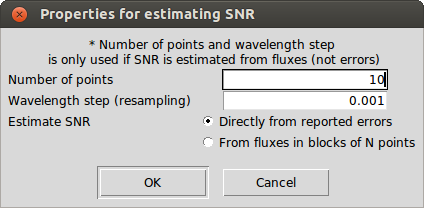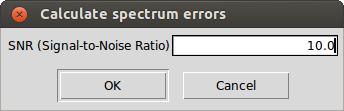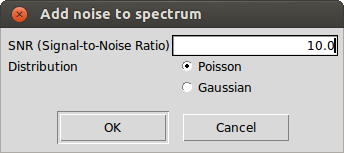# Flux errors

## Signal-to-Noise Ratio estimation

iSpec provides two different approaches to estimate the spectrum's signal-to-noise ratio (S/N) via "Parameters - Estimate SNR":• From errors: The S/N is calculated by using the flux divided by the reported errors in the spectrum. This is the best way to calculate the S/N if the errors are present.
• From fluxes: The whole spectrum is checked, resampling to ensure homogeneous steps and taking 10 by 10 measurements (although this value can be modified by the user), calculating the S/N for each one and finally selecting the mean S/N as the global S/N. The Signal-to-Noise Ratio (S/N) can be defined as the ratio of mean to standard deviation of a measurement:

$S/N = \frac{\mu}{\sigma}$

where $$\mu$$ is the mean value and $$\sigma$$ the standard deviation.

An estimation based on the errors (if they are included in the original observation) is more trustworthy than based on fluxes, since the latter will be influenced also by the stellar type. S/N estimations from fluxes should only be considered as relative values when comparing two spectra of similar spectral types where both S/N have been estimated similarly.

## Errors estimation

Given a S/N, iSpec can compute the errors associated to each flux measurement (in case they were not already reported in the original observation). This functionality is found in "Operations - Estimate errors based on S/N".Once executed, the errors can be plot via "Spectra - Show errors in plot".